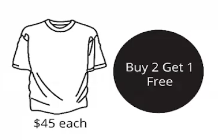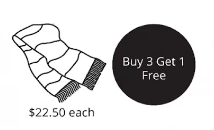Study P3 Mathematics Whole Numbers 3 - Geniebook# Whole Numbers 3

1. Whole Number Strategy – Grouping

## 1. Whole Number Strategy – Grouping

In  Whole Number Strategy – Grouping, we will solve questions by grouping the items in a group and determine the number of groups involved.

Question 1:

$$3$$ apples cost $$5$$. What is the cost of $$9$$ apples?Solution:

Number of apples in 1 group $$= 3$$ apples
Number of groups of apples\begin{align}\\ &= 9 \div 3\\ &= 3 \end{align}

Cost of 1 group of apples $$= 5$$
Cost of 3 groups of apples\begin{align}\\ &= 3 \times 5\\ &= 15 \end{align}

The cost of $$9$$ apples is $$15$$

$$15$$

Question 2:

A fruit seller sold $$5$$ mangoes for $$8$$. Marissa bought $$25$$ mangoes from the fruit seller. How much did she pay?

Solution:

Number of mangoes in 1 group $$= 5$$ mangoes
Number of groups of mangoes\begin{align}\\ &= 25 \div 5\\ &= 5 \end{align}

Cost of 1 group of mangoes $$= 8$$
Cost of 5 group of mangoes\begin{align}\\ &= 5 \times 8\\ &= 40 \end{align}

She paid $$40$$.

$$40$$

Question 3:

At a supermarket, every $$5$$ apples cost $$2$$ and every $$10$$ oranges cost $$3$$.. Find the total cost of $$10$$ apples and $$30$$ oranges.

Solution:

Number of apples in 1 group $$= 5$$ apples
Number of groups of apples\begin{align}\\ &= 10 \div 5\\ &= 2 \end{align}

Cost of 1 group of apples $$= 2$$
Cost of 2 groups of apples\begin{align}\\ &= 2 \times 2\\ &= 4 \end{align}

Number of oranges in 1 group $$= 10$$ oranges
Number of groups of oranges\begin{align}\\ &= 30 \div 10\\ &= 3 \end{align}

Cost of 1 group of oranges $$= 3$$
Cost of 3 groups of oranges\begin{align}\\ &= 3 \times 3\\ &= 9 \end{align}

Total cost of $$10$$ apples and $$30$$ oranges\begin{align}\\ &= 4 + 9\\ &= 13 \end{align}

The total cost of $$10$$ apples and $$30$$ oranges is $$13$$

$$13$$

Question 4:

Ahmad painted $$30$$ balls using either green or red paint. For every $$4$$ balls painted green, $$2$$ balls were painted red. How many balls were painted red?

Solution:

There are $$4$$ green balls and $$2$$ red balls red in 1 group.
Number of groups of balls\begin{align}\\ &= 30 \div 6\\ &= 5 \end{align}

Number of balls painted red in 1 group $$= 2$$
Number of red balls in 5 groups\begin{align}\\ &= 5 \times 2\\ &= 10 \end{align}

$$10$$ balls were painted red.

$$10$$ balls

Question 5:

Yvonne received $$3$$ free packets of milk for every $$5$$ packets of biscuits she bought. Given that she received $$27$$ free packets of milk, how many packets of biscuits did she buy?

Solution:

There are $$5$$ packets of biscuits and $$3$$ free packets of milk in 1 group.
Number of groups\begin{align}\\ &= 27 \div 3\\ &= 9 \end{align}

Number of packets of biscuits in 1 group $$= 5$$
Number of packets of biscuits in 9 groups\begin{align}\\ &= 9 \times 5\\ &= 45 \end{align}

She bought $$45$$ packets of biscuits.

$$45$$ packets

Question 6:

Helen received $$3$$ bonus stamps for every $$5$$ she spent in a supermarket. Given that she spent a total of $$189$$, how many bonus stamps will she get?

Solution:

Number of groups of $$5$$ in \begin{align}\\ 189 &= 189 \div 5\\ &= 37 \text{ groups R } 4 \end{align}

There are $$37$$ groups of $$5$$ and a remainder of $$4$$

Number of bonus stamps she will get\begin{align}\\ &= 37 \times 3\\ &= 111 \end{align}

She will get $$111$$ bonus stamps.

$$111$$ cookies

Question 7:

Pauline baked $$498$$ cookies to sell. She packed the cookies into boxes of 8 and sold each box for $$7$$. If she sold all the boxes of cookies, how much money did she collect?

Solution:

Number of boxes of $$8$$ cookies\begin{align}\\ &= 498 \div 8\\ &= 62 \text{ groups R 2 cookies} \end{align}

She packed $$62$$ boxes of $$8$$ cookies and there are $$2$$ cookies left.

Amount of money she collected\begin{align}\\ &= 62 \times 7\\ &= 434 \end{align}

She collected $$434$$

$$434$$

Question 8:

During a sale, notebooks were sold at $$5$$ for $$7$$. Given that Janice has $$50$$, what is the greatest number of notebooks she could buy?

Solution:

Number of groups of $$7$$ in \begin{align}\\ 50 &= 50 \div 7\\ &= 7 \text{ groups R } 1 \end{align}

There are $$7$$ groups of $$7$$ and a remainder of $$1$$.
Greatest number of notebooks she could buy\begin{align}\\ &= 7 \times 5\\ &= 35 \end{align}

The greatest number of notebooks she could buy is $$35$$

$$35$$

Question 9:

Tom has $$20$$. He wants to buy as many pens as he can. A pen costs $$2$$ while a pack of $$5$$ pens costs $$8$$. What is the greatest number of pens he could buy?

Solution:

Number of pens in 1 group $$= 5$$ pens

Cost of 1 group of pens $$= 8$$

Number of groups of $$8$$ in \begin{align}\\ 20 &= 20 \div 8\\ &= 2 \text{ groups R } 4 \end{align}

There are 2 groups of $$8$$ and a remainder of $$4$$

Number of pens in 2 groups\begin{align}\\ &= 2 \times 5\\ &= 10 \end{align}

Cost of 1 pen $$= 2$$

Number of pens Tom can buy with the remaining \begin{align}\\ 4 &= 4 \div 2\\ &= 2 \end{align}

Greatest number of pens Tom could buy\begin{align}\\ &= 10 + 2\\ &= 12 \end{align}

The greatest number of pens Tom could buy is $$12$$

$$12$$

Question 10:

A fruit seller bought $$17$$ boxes of mangoes. Each box contained $$5$$ mangoes. He repacked the mangoes into packets of $$3$$ and sold all the packets at $$10$$ each. How much money did he collect?

Solution:

Total number of mangoes\begin{align}\\ &= 17 \times 5\\ &= 85 \end{align}

Number of packets of $$3$$ mangoes\begin{align}\\ &= 85 \div 3\\ &= 28 \text{ groups R } 1 \text{ mango} \end{align}

The fruit seller packed $$28$$ packets of $$3$$ mangoes and had $$1$$ mango left.

Cost of 1 packet of mangoes $$= 10$$

Amount of money he collected from $$28$$ packets of mangoes\begin{align}\\ &= 28 \times 10\\ &= 280 \end{align}

He collected $$280$$ from his sale.

$$280$$

Question 11:

The following shows the ongoing promotion for T-shirts at a store.Terry wants to get $$12$$ T-shirts. What is the least amount of money he has to pay?

Solution:

Number of T-shirts in 1 group\begin{align}\\ &= 2 + 1\\ &= 3 \end{align}
Number of groups of 3 T-shirts\begin{align}\\ &= 12 \div 3\\ &= 4 \end{align}
Cost of 1 group of T-shirts\begin{align}\\ &= 2 \times 45\\ &= 90 \end{align}
Cost of 4 groups of T-shirts\begin{align}\\ &= 4 \times 90\\ &= 360 \end{align}

The least amount he has to pay is $$360$$

$$360$$

Question 12:

The following shows the ongoing promotion for scarves at a store.Violet wants to buy $$11$$ scarves.  What is the least amount of money she has to pay?

Solution:

Number of scarves in 1 group\begin{align}\\ &= 3 + 1\\ &= 4 \end{align}
Number of groups of 4 scarves\begin{align}\\ &= 11 \div 4\\ &= 2 \text{ groups R } 3 \text{ scarves} \end{align}

To get $$11$$ scarves, there are 2 groups of $$4$$ scarves and another $$3$$ scarves.

Number of free scarf in 1 group $$= 1$$
Number of free scarves in 2 groups\begin{align}\\ &= 2 \times 1\\ &= 2 \end{align}

Number of scarves paid for\begin{align}\\ &= 11-2\\ &= 9 \end{align}

Total amount of money Violet need to pay\begin{align}\\ &= 9 \times 22.50\\ &= 202.50 \end{align}

Violet needs to pay $$202.50$$

$$202.50$$

Question 13:

Gary bought $$15$$ donuts at Shop A. Aziz spent the same amount of money as Gary at Shop B. How many donuts did Aziz buy at Shop B?Solution

Gary (Shop A):

Number of donuts in 1 group $$= 3$$

Number of groups of donuts Gary bought\begin{align}\\ &= 15 \div 3\\ &= 5 \end{align}

Cost of 1 group of donuts $$= 2$$

Cost of 5 groups of donuts\begin{align}\\ &= 5 \times 2\\ &= 10 \end{align}

Since Aziz spent the same amount of money as Gary, Aziz spent $$10$$ on donuts at Shop B.

Aziz (Shop B):

Number of groups of $$5$$ in \begin{align}\\ 10 &= 10 \div 5\\ &= 2 \end{align}

Number of donuts in 1 group $$= 6$$

Number of donuts in 2 groups\begin{align}\\ &= 2 \times 6\\ &= 12 \end{align}

Aziz bought $$12$$ donuts at Shop B.

12 donuts

Continue Learning
Volume Money
Mass Fractions
Addition And Subtraction Whole Numbers 1
Whole Numbers 3 Whole Numbers 6
Bar Graphs Angles
Multiplication and Division Length, Mass And Volume
Length Area and Perimeter
Parallel And Perpendicular LinesPrimaryPrimary 1Primary 2Primary 3EnglishMaths
Volume
Money
Mass
Fractions
Whole Numbers 1
Whole Numbers 3
Whole Numbers 6
Bar Graphs
Angles
Multiplication and Division
Length, Mass And Volume
Length
Area and Perimeter
Parallel And Perpendicular LinesSciencePrimary 4Primary 5Primary 6SecondarySecondary 1Secondary 2Secondary 3Secondary 4